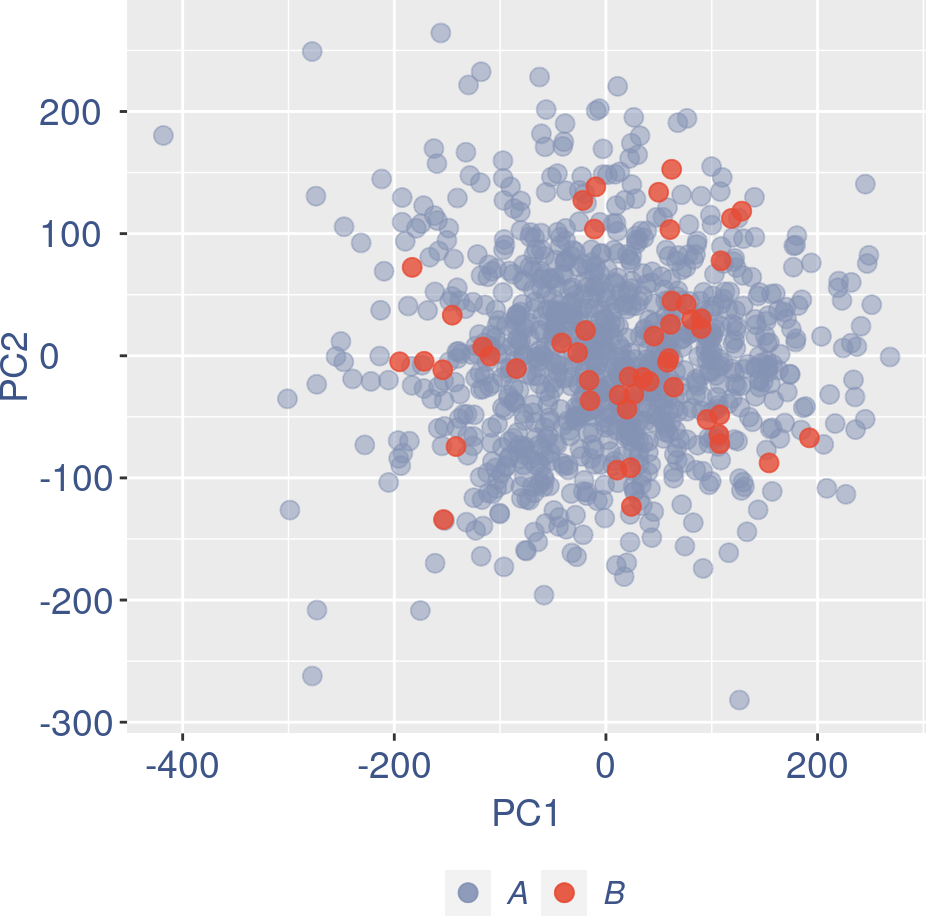# Contents

``````library(COTAN)
library(data.table)
library(Matrix)
library(ggrepel)
library(factoextra)
#> Welcome! Want to learn more? See two factoextra-related books at https://goo.gl/ve3WBa
library(Rtsne)
library(utils)
library(plotly)
#>
#> Attaching package: 'plotly'
#> The following object is masked from 'package:ggplot2':
#>
#>     last_plot
#> The following object is masked from 'package:stats':
#>
#>     filter
#> The following object is masked from 'package:graphics':
#>
#>     layout
library(tidyverse)
#> ── Attaching packages
#> ───────────────────────────────────────
#> tidyverse 1.3.2 ──
#> ✔ tibble  3.1.8      ✔ dplyr   1.0.10
#> ✔ tidyr   1.2.1      ✔ stringr 1.4.1
#> ✔ readr   2.1.3      ✔ forcats 0.5.2
#> ✔ purrr   0.3.5
#> ── Conflicts ────────────────────────────────────────── tidyverse_conflicts() ──
#> ✖ dplyr::filter()    masks plotly::filter(), stats::filter()
library(htmlwidgets)
library(MASS)
#>
#> Attaching package: 'MASS'
#>
#> The following object is masked from 'package:dplyr':
#>
#>     select
#>
#> The following object is masked from 'package:plotly':
#>
#>     select
library(dendextend)
#>
#> ---------------------
#> Welcome to dendextend version 1.16.0
#> Type citation('dendextend') for how to cite the package.
#>
#> Type browseVignettes(package = 'dendextend') for the package vignette.
#> The github page is: https://github.com/talgalili/dendextend/
#>
#> Suggestions and bug-reports can be submitted at: https://github.com/talgalili/dendextend/issues
#> You may ask questions at stackoverflow, use the r and dendextend tags:
#>   https://stackoverflow.com/questions/tagged/dendextend
#>
#>  To suppress this message use:  suppressPackageStartupMessages(library(dendextend))
#> ---------------------
#>
#>
#> Attaching package: 'dendextend'
#>
#> The following object is masked from 'package:data.table':
#>
#>     set
#>
#> The following object is masked from 'package:stats':
#>
#>     cutree``````
``````mycolours <- c("A" = "#8491B4B2","B"="#E64B35FF")
my_theme = theme(axis.text.x = element_text(size = 14, angle = 0, hjust = .5, vjust = .5, face = "plain", colour ="#3C5488FF" ),
axis.text.y = element_text( size = 14, angle = 0, hjust = 0, vjust = .5, face = "plain", colour ="#3C5488FF"),
axis.title.x = element_text( size = 14, angle = 0, hjust = .5, vjust = 0, face = "plain", colour ="#3C5488FF"),
axis.title.y = element_text( size = 14, angle = 90, hjust = .5, vjust = .5, face = "plain", colour ="#3C5488FF"))
data_dir = tempdir()``````

This is just an example on a toy dataset. If the user want to try on a real dataset it can be used the previous command to download it and the next commented line to use it as input data. There are also available online many other examples at link.

``````data("ERCCraw", package = "COTAN")

ERCCraw = as.data.frame(ERCCraw)
rownames(ERCCraw) = ERCCraw\$V1
ERCCraw = ERCCraw[,2:ncol(ERCCraw)]
ERCCraw[1:5,1:5]
#>            AAACCGTGAAGCCT.1 AAACGCACTGCTGA.1 AAACTTGACCGAAT.1 AAAGACGAGGAAGC.1
#> ERCC-00002             1662             4036             3919             4124
#> ERCC-00003               95              283              298              290
#> ERCC-00004               25               75               70               75
#> ERCC-00009               41               57               78               98
#> ERCC-00012                0                0                0                0
#>            AAAGACGATCCGAA.1
#> ERCC-00002             4220
#> ERCC-00003              312
#> ERCC-00004               87
#> ERCC-00009               87
#> ERCC-00012                0``````

Define a directory where the output will be stored.

``out_dir <- paste0(tempdir(),"/")``

# 1 Analytical pipeline

Inizialise the COTAN object with the row count table and the metadata for the experiment.

``````obj = new("scCOTAN",raw = ERCCraw)
#obj = initRaw(obj,GEO="GSM2861514" ,sc.method="Drop_seq",cond = "mouse cortex E17.5")
obj = initRaw(obj,GEO="ERCC" ,sc.method="10X",cond = "negative ERCC dataset")
#>  "Initializing S4 object"``````

Now we can start the cleaning. Analysis requires and starts from a matrix of raw UMI counts after removing possible cell doublets or multiplets and low quality or dying cells (with too high mtRNA percentage, easily done with Seurat or other tools).

If we do not want to consider the mitochondrial genes we can remove them before starting the analysis.

``````genes_to_rem = get.genes(obj)[grep('^mt', get.genes(obj))]
cells_to_rem = names(get.cell.size(obj)[which(get.cell.size(obj) == 0)])
obj = drop.genes.cells(obj,genes_to_rem,cells_to_rem )``````

We want also to define a prefix to identify the sample.

``````#t = "E17.5_cortex"
t = "ERCC"

print(paste("Condition ",t,sep = ""))
#>  "Condition ERCC"
#--------------------------------------
n_cells = length(get.cell.size(object = obj))
print(paste("n cells", n_cells, sep = " "))
#>  "n cells 1015"

n_it = 1``````

## 1.1 Data cleaning

First we create the directory to store all information regarding the data cleaning.

``````if(!file.exists(out_dir)){
dir.create(file.path(out_dir))
}

if(!file.exists(paste(out_dir,"cleaning", sep = ""))){
dir.create(file.path(out_dir, "cleaning"))
}``````
``````ttm = clean(obj)
#>  "Start estimation mu with linear method"
#>    88 1015
#>  "Start PCA"
#>  "starting hclust"

obj = ttm\$object

ttm\$pca.cell.2``````Run this when B cells need to be removed.

``````pdf(file.path(out_dir,"cleaning",paste(t,"_",n_it,"_plots_before_cells_exlusion.pdf", sep = "")))
ttm\$pca.cell.2
ggplot(ttm\$D, aes(x=n,y=means)) + geom_point() +
geom_text_repel(data=subset(ttm\$D, n > (max(ttm\$D\$n)- 15) ), aes(n,means,label=rownames(ttm\$D[ttm\$D\$n > (max(ttm\$D\$n)- 15),])),
nudge_y      = 0.05,
nudge_x      = 0.05,
direction    = "x",
angle        = 90,
vjust        = 0,
segment.size = 0.2)+
ggtitle("B cell group genes mean expression")+my_theme +
theme(plot.title = element_text(color = "#3C5488FF", size = 20, face = "italic",vjust = - 5,hjust = 0.02 ))
dev.off()

if (length(ttm\$cl1) < length(ttm\$cl2)) {
to_rem = ttm\$cl1
}else{
to_rem = ttm\$cl2
}
n_it = n_it+1
obj = drop.genes.cells(object = obj,genes = c(),cells = to_rem)

gc()

ttm = clean(obj)
#ttm = clean.sqrt(obj, cells)
obj = ttm\$object

ttm\$pca.cell.2
``````

Run this only in the last iteration, instead the previous code, when B cells group has not to be removed

``````pdf(file.path(out_dir,"cleaning",paste(t,"_",n_it,"_plots_before_cells_exlusion.pdf", sep = "")))
ttm\$pca.cell.2
ggplot(ttm\$D, aes(x=n,y=means)) + geom_point() +
geom_text_repel(data=subset(ttm\$D, n > (max(ttm\$D\$n)- 15) ), aes(n,means,label=rownames(ttm\$D[ttm\$D\$n > (max(ttm\$D\$n)- 15),])),
nudge_y      = 0.05,
nudge_x      = 0.05,
direction    = "x",
angle        = 90,
vjust        = 0,
segment.size = 0.2)+
ggtitle(label = "B cell group genes mean expression", subtitle = " - B group NOT removed -")+my_theme +
theme(plot.title = element_text(color = "#3C5488FF", size = 20, face = "italic",vjust = - 10,hjust = 0.02 ),
plot.subtitle = element_text(color = "darkred",vjust = - 15,hjust = 0.01 ))

dev.off()
#> png
#>   2``````

To color the pca based on nu_j (so the cells’ efficiency)

``````nu_est = round(get.nu(object = obj), digits = 7)

plot.nu <-ggplot(ttm\$pca_cells,aes(x=PC1,y=PC2, colour = log(nu_est)))

plot.nu = plot.nu + geom_point(size = 1,alpha= 0.8)+
scale_color_gradient2(low = "#E64B35B2",mid =  "#4DBBD5B2", high =  "#3C5488B2" ,
midpoint = log(mean(nu_est)),name = "ln(nu)")+
ggtitle("Cells PCA coloured by cells efficiency") +
my_theme +  theme(plot.title = element_text(color = "#3C5488FF", size = 20),
legend.title=element_text(color = "#3C5488FF", size = 14,face = "italic"),
legend.text = element_text(color = "#3C5488FF", size = 11),
legend.key.width = unit(2, "mm"),
legend.position="right")

pdf(file.path(out_dir,"cleaning",paste(t,"_plots_PCA_efficiency_colored.pdf", sep = "")))
plot.nu
dev.off()
#> png
#>   2

plot.nu``````